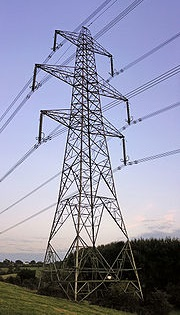#### You may also likeMake a set of numbers that use all the digits from 1 to 9, once and once only. Add them up. The result is divisible by 9. Add each of the digits in the new number. What is their sum? Now try some other possibilities for yourself!### Counting Factors

Is there an efficient way to work out how many factors a large number has?### Repeaters

Choose any 3 digits and make a 6 digit number by repeating the 3 digits in the same order (e.g. 594594). Explain why whatever digits you choose the number will always be divisible by 7, 11 and 13.

##### Age 11 to 14Challenge Level

Powers of numbers behave in surprising ways...

Take a look at the following and try to explain what's going on.Work out $2^1, 2^2, 2^3, 2^4, 2^5, 2^6$...

For which values of $n$ will $2^n$ be a multiple of $10?$

For which values of $n$ is $1^n + 2^n + 3^n$ even?

Work out $1^n + 2^n + 3^n + 4^n$ for some different values of $n$.

What do you notice?

What about $1^n + 2^n + 3^n + 4^n + 5^n?$

What other surprising results can you find?

Here are some suggestions to start you off:

$4^n + 5^n + 6^n$
$2^n+3^n$ for odd values of $n$
$3^n + 8^n$
$2^n + 4^n + 6^n$
$3^n + 5^n + 7^n$
$3^n - 2^n$
$7^n + 5^n - 3^n$